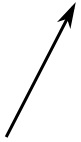# Linear Algebra

Machine Learning experts cannot live without Linear Algebra:

• ML make heavy use of Scalars
• ML make heavy use of Vectors
• ML make heavy use of Matrices
• ML make heavy use of Tensors

The purpose of this chapter is to highlight the parts of linear algebra that is used in data science projects like machine learning and deep learning.

ScalarVector(s)
1
 1 2 3

 1 2 3

MatrixTensor
 1 2 3 4 5 6

 1 2 3 4 5 6

 4 5 6 1 2 3

## Vectors and Matrices

Vectors and Matrices are the languages of data.

With ML, most things are done with vectors and matrices.

With vectors and matrices, you can Discover Secrets.

## Scalars

In linear algebra, a scalar is a single number.

In JavaScript it can be written like a constant or a variable:

const myScalar = 1;
let x = 1;
var y = 1;

## Vectors

In linear algebra, a vector is an array of numbers.

In JavaScript, it can be written as an array:

const myArray = [50,60,70,80,90,100,110,120,130,140,150];
myArray.length;   // the length of myArray is 11
Try it Yourself »

An array can have multiple dimensions, but a vector is a 1-dimensional array.

A vector can be written in many ways. The most common are:

v =
 1 2 3

or:

v =
 1 2 3The image to the left is a Vector. The Length shows the Magnitude. The Arrow shows the Direction.

## Matrices

In linear algebra, a matrix is a 2-dimensional array.

C =
 3 0 0 0 0 3 0 0 0 0 3 0 0 0 0 3

In JavaScript, a matrix is an array with 2 indices (indexes).

### Example

const myArray = [[1,2],[3,4],[5,6]];
Try it Yourself »

## Tensors

A Tensor is an N-dimensional Matrix.

T =

 1 2 3 4 5 6

 4 5 6 1 2 3

In JavaScript, a tensor is an array with multiple indices (indexes).